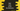# Different ways to find the sum of all dart list elements# Introduction :

Lists are iterable in dart. Lists are categorized into two kinds: growable and fixed-length list. We can change the size of a growable list and for fixed-length lists, the size is fixed. Sometimes, we need to find the total sum of all elements of an integer list. We can easily do that by iterating through each element of the list.

But dart provides a couple of methods to find out the sum of all elements without using a loop. In this post, I will show you different ways to find out the sum of all elements of a list. Let’s have a look.

## Method 1: Using a loop :

This is the most commonly used method. Iterate through the list using a loop and add all elements of the list to a final sum variable. We are using one for loop here :

``````main(List<String> args) {
var sum = 0;
var given_list = [1, 2, 3, 4, 5];

for (var i = 0; i < given_list.length; i++) {
sum += given_list[i];
}

print("Sum : \${sum}");
}``````

## Method 2: Using forEach :

forEach is another way to iterate through a list. We can also use this method to find out the total sum of all values in a dart list. It is similar to the above method. The only difference is that we don’t have to initialize another variable i and list.length is not required.

``````main(List<String> args) {
var sum = 0;
var given_list = [1, 2, 3, 4, 5];

given_list.forEach((e) => sum += e);

print("Sum : \${sum}");
}``````

Much simpler than using a for loop.

## Method 3: Using reduce :

reduce method combines all elements of a list iteratively to one single value using a given function. We can use this method to find out the sum of all elements as like below :

``````main(List<String> args) {
var given_list = [1, 2, 3, 4, 5];

var sum = given_list.reduce((value, element) => value + element);

print("Sum : \${sum}");
}``````

Note that reduce will fail if the list is empty.

## Method 4: Using fold :

fold() is similar to reduce. It combines all elements of a list iteratively to one single value using a function. It takes one initial value and calculates the final value based on the previous value.

``````main(List<String> args) {
var sum = 0;
var given_list = [1,2,3,4,5];

sum = given_list.fold(0, (previous, current) => previous + current);

print("Sum : \${sum}");
}``````

Using fold, we can find out the sum of an empty list.

### Combining all methods :

``````main(List<String> args) {
var sum = 0;
var given_list = [1, 2, 3, 4, 5];
for (var i = 0; i < given_list.length; i++) {
sum += given_list[i];
}

print("Sum (for loop): \${sum}");

sum = 0;
given_list.forEach((e) => sum += e);
print("Sum (forEach): \${sum}");

sum = given_list.reduce((value, element) => value + element);
print("Sum (reduce): \${sum}");

sum = given_list.fold(0, (previous, current) => previous + current);
print("Sum (fold): \${sum}");
}``````